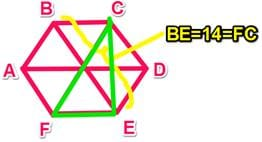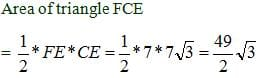Directions: Each GMAT Data Sufficiency proble...

### Related TestDirections: Each GMAT Data Sufficiency problem consists of a question and two statements labeled (1) and (2), that provide data. Based on the data given plus your knowledge of mathematics and everyday facts, you must decide whether the data are sufficient for answering the question. The five answer choices are the same for every data sufficiency question.
What is the area of a triangle (with vertices at FCE) that is inscribed in a hexagon with vertices at ABCDE?
(1) The hexagon is regular and BE = 14.
(2) EC = 7√3.
• a)
Statement (1) ALONE is sufficient, but statement (2) alone is not sufficient to answer thequestion asked;
• b)
Statement (2) ALONE is sufficient, but statement (1) alone is not sufficient to answer thequestion asked;
• c)
BOTH statements (1) and (2) TOGETHER are sufficient to answer the question asked,but NEITHER statement ALONE is sufficient;
• d)
• e)
Statements (1) and (2) TOGETHER are NOT sufficient to answer the question asked,and additional data are needed.EduRev GMAT
Statement 1:A regular hexagon can be divided into six equilateral triangles, each with a central angle measure of 60 degrees. Remember that we can inscribe a regular hexagon in a circle such that each vertex is a point on the circumference of the circle. Central Angle Theorem states that the central angle in a circle is always twice an inscribed angle when the point of the inscribed angle (in this case point C) is on the major arc. For this figure, this means angle FCE = 30. Since angle CFE = 60, we have a 30-60-90 triangle. If BE = 14, then FC = 14 as well. We’d now be able to solve for the height (CE) and base (FE) using the 30-60-90 side ratios. Since this is a data sufficiency question, we can stop here and conclude that statement 1 is sufficient.
However, if you actually had to calculate the area of triangle FCE, here is how you might proceed: The sides of a 30-60-90 are in the ratio 1 : √3 : 2 , so the sides of triangle FCE will have the following lengths: FE = 7, CE= 7√3 , FC=14.Statement 2:
If the hexagon is regular, we can calculate the area of triangle FCE using a process similar to the one illustrated above. However, statement 2 does not tell us that the hexagon is regular. If the hexagon is not regular, we can come up with different areas for triangle FCE, depending on what we assume. Statement 2 alone is not sufficient.View courses related to this question Explore GMAT courses
 Explore GMAT coursesView courses related to this question1 Crore+ students have signed up on EduRev. Have you?

• ### Directions: Each Data Sufficiency problem consists of a question and two st... more(Scan QR code)